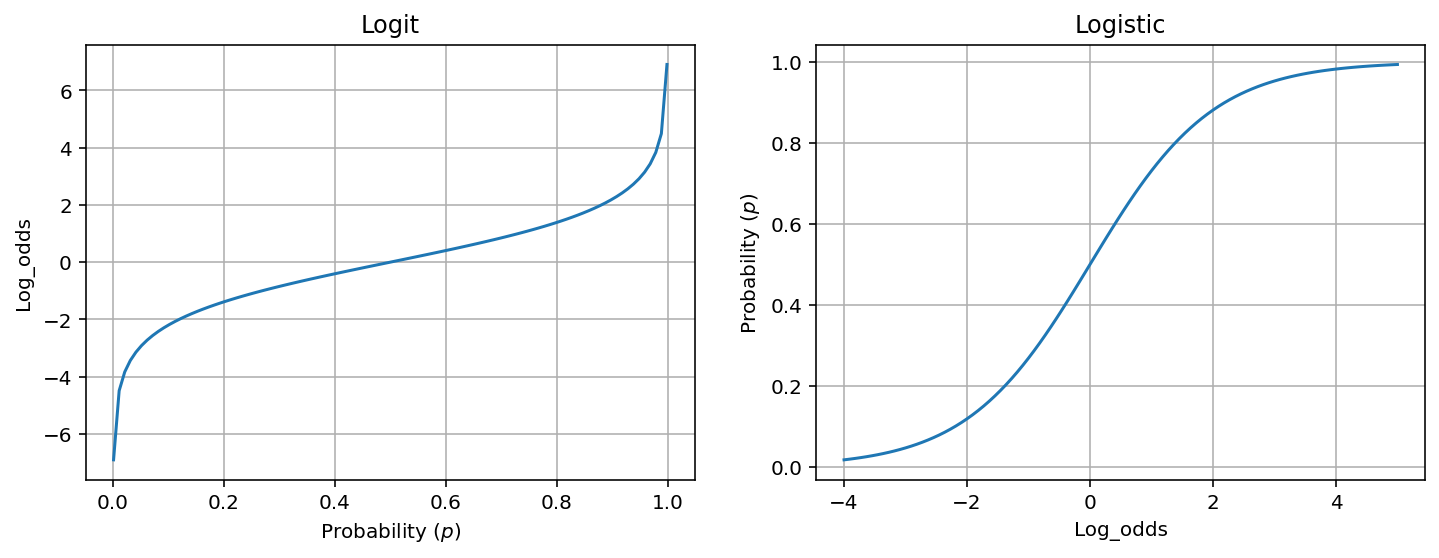Here, I list some functions related to logistic regression that I get confused often.

# Logit

Logit function is basically the log of odds:

\begin{align} \text{logit}(p) = \log \frac{p}{1-p} \end{align}

where $p$ is a probability.

# Logistic function

Logistic function is a common example of sigmoid function, which produces a S-shaped curve (aka. sigmoid curve).

\begin{align} f(z) = \frac{e^{z}}{1 + e^{z}} = \frac{1}{1 + e^{-z}} \end{align}

Some simple algebra shows that the logistic function is the inverse of the logit function (we use $p$ instead of $f(z)$ to be more intuitive as it relates to probability):

\begin{align} p &= \frac{e^z}{1 + e^z} \\ p + p e^z &= e^z \\ e^z &= \frac{p}{1-p} \\ z &= \log \frac{p}{1-p} \\ \end{align}

Note, $f(z)$ is commonly interpreted as a probability as it ranges from 0 (when $z \rightarrow -\infty$) to 1 (when $z \rightarrow \infty$).

In logistic regression, we are basically using a linear function of features to estimate the $z$, the log odds.Figure 1. Plots of logit and Logistic functions. Note the axes are reversed between logit and logistic plots as they are inverse function of each other (Notebook).

# Softmax function

Softmax function is the extension of logistic function to more than two dimensions.

Suppose $\mathbf{z} = (z_1, \cdots, z_d) \in \mathbb{R}^d$.

\begin{align} f(z_k) = \frac{e^{z_k}}{\sum_{i=1}^{K} e^{z_k}} \end{align}

If d dimensions correspond to d categories, then each $f(z_k)$ can be interpreted as the probability of category $i$.

Note, adding a constant ($C$) to each $z_k$ won’t affect $f(z_k)$:

\begin{align} \frac{e^{z_k + C}}{\sum_{i=1}^{K} e^{z_k + C}} = \frac{e^C e^{z_k}}{e^C \sum_{i=1}^{K} e^{z_k}} = \frac{e^{z_k}}{\sum_{i=1}^{K} e^{z_k}} \end{align}

so for mathematical convenience, we can offset all components in $\mathbf{z}$ so that $z_1 = 0$. Then in the case of 2 dimension, softmax function simplifies to the logistic function.

# Multinomial logit function

From the softmax function, we could derive the corresponding logit function for more than two dimensions.

\begin{align} p(z_k) &= \frac{e^{z_k}}{\sum_{i=1}^{K} e^{z_k}} \\ p(z_k) &= \frac{e^{z_k}}{e^{z_k} + \sum_{i \ne k}^{K} e^{z_k}} \\ p(z_k)e^{z_k} + p(z_k) \sum_{i \ne k}^{K} e^{z_k} &= e^{z_k} \\ e^{z_k} &= \frac{p(z_k) \sum_{i \ne k}^{K} e^{z_k} }{1 - p(z_k)} \\ z_k &= \log \frac{p(z_k) \sum_{i \ne k}^{K} e^{z_k} }{1 - p(z_k)} \end{align}

So $z_k$ is a log-odds weighted by $\sum_{i \ne k}^{K} e^{z_k}$.